## Access Answers to NCERT Class 7 Maths Chapter 15 – Visualising Solid Shapes Exercise 15.3

### Access Answers to NCERT Class 7 Maths Chapter 15 – Visualising Solid Shapes Exercise 15.3

1. What cross-sections do you get when you give a

(i) vertical cut (ii) horizontal cut

to the following solids?

(a) A brick

Solution:-

The cross-section of a brick when it is cut into vertically is as shown in the figure below,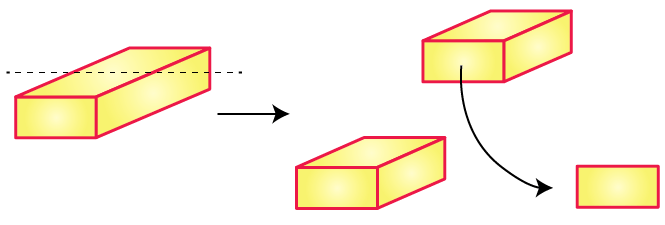The cross-section of a brick when it is cut into horizontally is as shown in the figure below,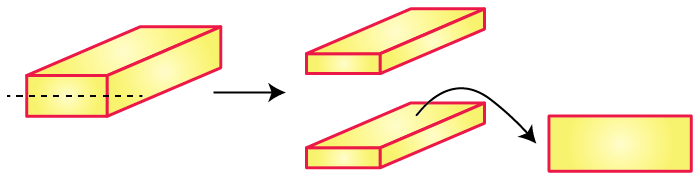(b) A round apple

Solution:-

The cross-section of a round apple when it is cut into vertically is as shown in the figure below,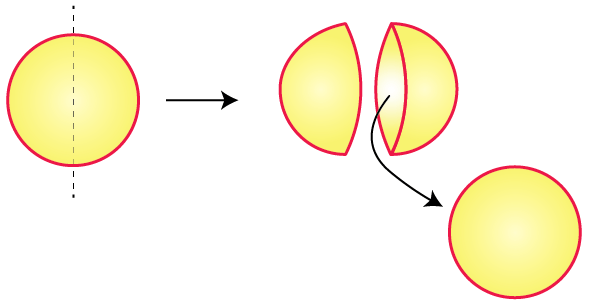The cross-section of a round apple when it is cut into horizontally is as shown in the figure below,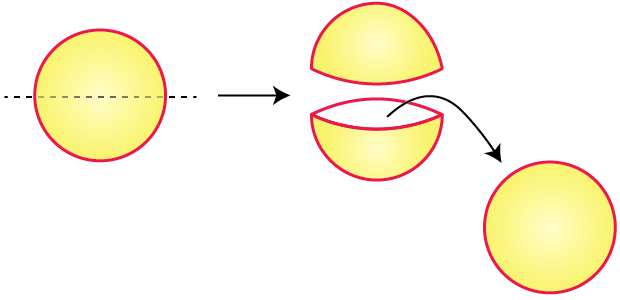(c) A die

Solution:-

The cross-section of a die when it is cut into vertically is as shown in the figure below,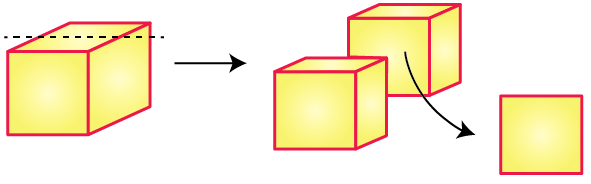The cross-section of a die when it is cut into horizontally is as shown in the figure below,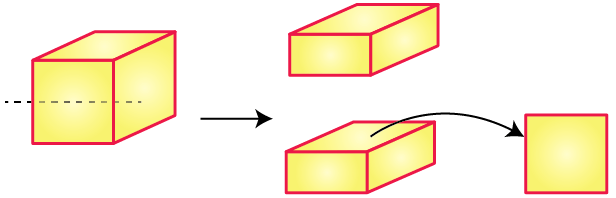(d) A circular pipe

Solution:-

The cross-section of a circular pipe when it is cut into vertically is as shown in the figure below,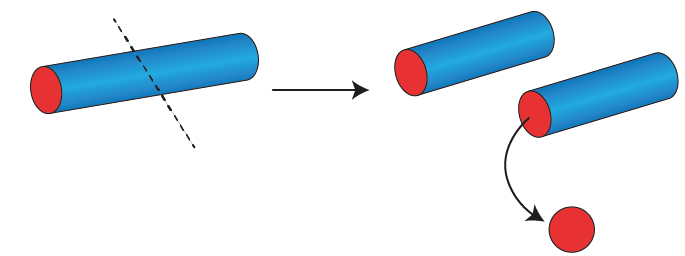The cross-section of a circular pipe when it is cut into horizontally is as shown in the figure below,(e) An ice cream cone

Solution:-

The cross-section of an ice cream when it is cut into vertically is as shown in the figure below,The cross-section of an ice cream when it is cut into horizontally is as shown in the figure below,